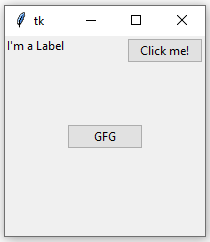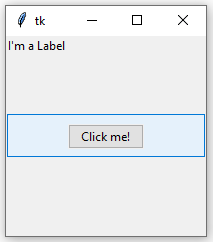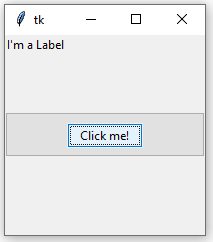# Python | place() method in Tkinter

The Place geometry manager is the simplest of the three general geometry managers provided in Tkinter. It allows you explicitly set the position and size of a window, either in absolute terms, or relative to another window.
You can access the place manager through the `place()` method which is available for all standard widgets.

It is usually not a good idea to use `place()` for ordinary window and dialog layouts; its simply to much work to get things working as they should. Use the `pack()` or `grid()` managers for such purposes.

Syntax:

```widget.place(relx = 0.5, rely = 0.5, anchor = CENTER)
```

Note : `place()` method can be used with `grid()` method as well as with `pack()` method.

Code #1:

 `# Importing tkinter module ` `from` `tkinter ``import` `*` `from` `tkinter.ttk ``import` `*` ` `  `# creating Tk window ` `master ``=` `Tk() ` ` `  `# setting geometry of tk window ` `master.geometry(``"200x200"``) ` ` `  `# button widget ` `b1 ``=` `Button(master, text ``=` `"Click me !"``) ` `b1.place(relx ``=` `1``, x ``=``-``2``, y ``=` `2``, anchor ``=` `NE) ` ` `  `# label widget ` `l ``=` `Label(master, text ``=` `"I'm a Label"``) ` `l.place(anchor ``=` `NW) ` ` `  `# button widget ` `b2 ``=` `Button(master, text ``=` `"GFG"``) ` `b2.place(relx ``=` `0.5``, rely ``=` `0.5``, anchor ``=` `CENTER) ` ` `  `# infinite loop which is required to ` `# run tkinter program infinitely ` `# until an interrupt occurs ` `mainloop() `

Output:When we use `pack()` or `grid()` managers, then it is very easy to put two different widgets separate to each other but putting one of them inside other is a bit difficult. But this can easily be achieved by `place()` method.
In place() method, we can use in_ option to put one widget inside other.

Code #2:

 `# Importing tkinter module ` `from` `tkinter ``import` `*` `from` `tkinter.ttk ``import` `*` ` `  `# creating Tk window ` `master ``=` `Tk() ` ` `  `# setting geometry of tk window ` `master.geometry(``"200x200"``) ` ` `  `# button widget ` `b2 ``=` `Button(master, text ``=` `"GFG"``) ` `b2.pack(fill ``=` `X, expand ``=` `True``, ipady ``=` `10``) ` ` `  `# button widget ` `b1 ``=` `Button(master, text ``=` `"Click me !"``) ` ` `  `# This is where b1 is placed inside b2 with in_ option ` `b1.place(in_``=` `b2, relx ``=` `0.5``, rely ``=` `0.5``, anchor ``=` `CENTER) ` ` `  `# label widget ` `l ``=` `Label(master, text ``=` `"I'm a Label"``) ` `l.place(anchor ``=` `NW) ` ` `  `# infinite loop which is required to ` `# run tkinter program infinitely ` `# until an interrupt occurs ` `mainloop() `

Output: In below images notice that one button is placed inside the other.My Personal Notes arrow_drop_upCheck out this Author's contributed articles.

If you like GeeksforGeeks and would like to contribute, you can also write an article using contribute.geeksforgeeks.org or mail your article to contribute@geeksforgeeks.org. See your article appearing on the GeeksforGeeks main page and help other Geeks.

Please Improve this article if you find anything incorrect by clicking on the "Improve Article" button below.

Article Tags :

Be the First to upvote.

Please write to us at contribute@geeksforgeeks.org to report any issue with the above content.